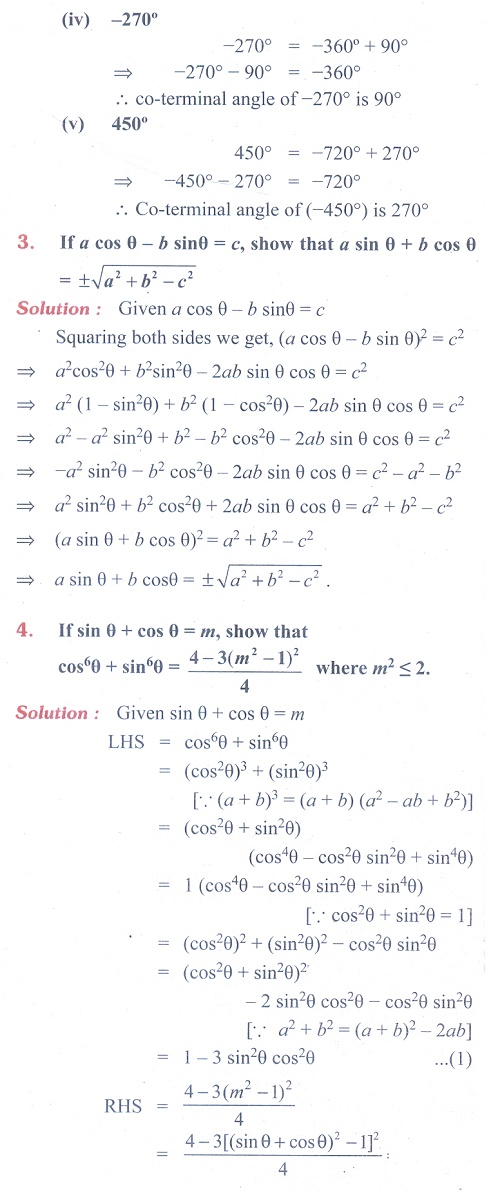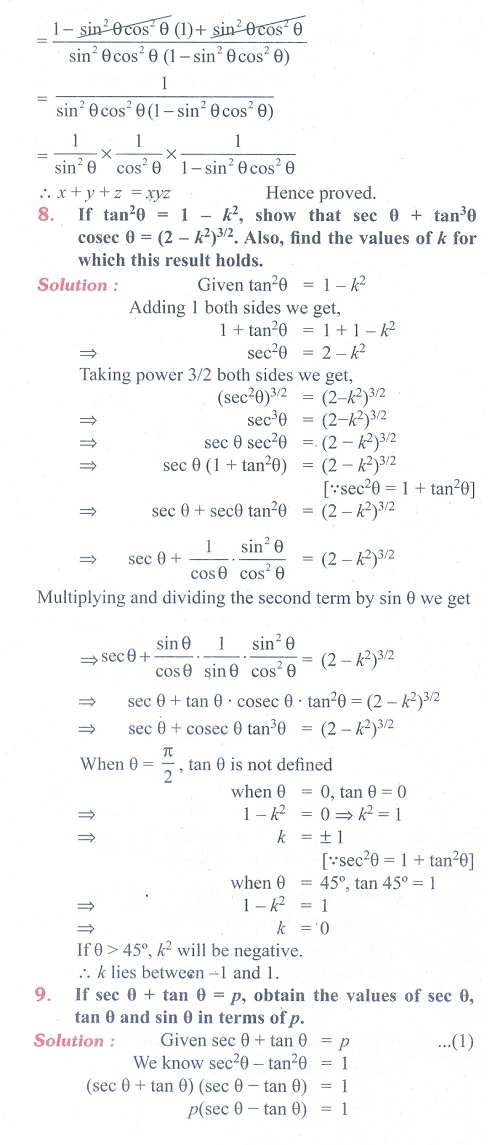Home | | Maths 11th std | Exercise 3.1: Basic results of Trigonometry

# Exercise 3.1: Basic results of Trigonometry

Maths Book back answers and solution for Exercise questions - Mathematics : Trigonometry: Basic results of TrigonometryTags : Problem Questions with Answer, Solution | Mathematics , 11th Mathematics : UNIT 3 : Trigonometry
Study Material, Lecturing Notes, Assignment, Reference, Wiki description explanation, brief detail
11th Mathematics : UNIT 3 : Trigonometry : Exercise 3.1: Basic results of Trigonometry | Problem Questions with Answer, Solution | Mathematics